## Classic Mathematical Books To Read

Aug 26, 2022

Here we have compiled the list of best mathematics books that will assist both the students and researchers who are buried in the daily grind in coming up with their next big discovery.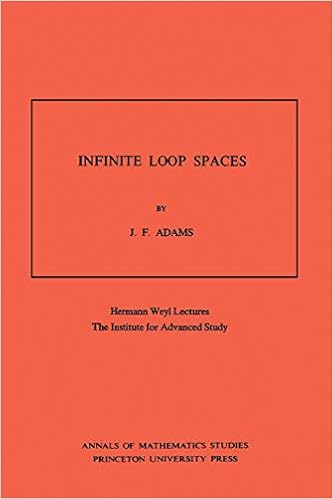### Book 1: Infinite Loop Spaces (AM-90), Volume 90: Hermann Weyl Lectures, The Institute for Advanced Study. (AM-90) (Annals of Mathematics Studies, 90) First Edition

Author: John Frank Adams Category: Geometry, Geometry & Topology

Current research in algebraic topology has mostly focused on the concept of infinite loop spaces. For scholars and students, Frank Adams provides a summary of this substantial effort. He does an excellent job of inspiring the audience. His colloquial writing style flows as seamlessly as a completely didactic text, yet it will offer you enough knowledge and analysis that it seems like you can fill in the information on your own if you want to.

The principal subjects included are: generalized cohomology theories and spectra; infinite-loop space machines in the sense of Boardman-Vogt, May, and Segal; localization and group completion; the transfer; the Adams conjecture and several proofs of it; and the recent theories of Adams and Priddy and of Madsen, Snaith, and Tornehave.

Buy this book at Amazon Book Store.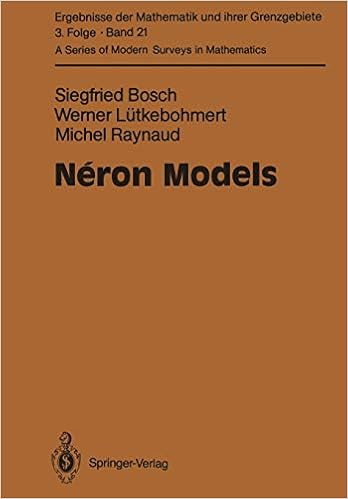### Book 2: Néron Models (Ergebnisse der Mathematik und ihrer Grenzgebiete. 3. Folge / A Series of Modern Surveys in Mathematics, 21) Softcover reprint of the original 1st ed. 1990 Edition

Author: Siegfried Bosch, Werner Lütkebohmert, Michel Raynaud Category: Algebraic Geometry, Geometry

Early in the 1960s, A. Néron developed Néron models to investigate the integral structure of abelian varieties over several fields. Since that day, the theory of Néron models has found widespread use among mathematicians and algebraic geometers.

Significant advancements in arithmetic algebraic geometry have sparked an interest in learning more about Néron models and even returning to the fundamentals of their formation. This has functioned as the authors' motivation to provide a detailed analysis of Néron models. The development of Néron models is thoroughly illustrated in this volume of the acclaimed "Ergebnisse" series from the perspective of Grothendieck's algebraic geometry. The book's second section explains how, in the case of Jacobian varieties, Néron models relate to the relative Picard functor. To help the reader, the authors remind them of a few significant standard algebraic geometry approaches. The Picard functor's theoretical foundations are also covered in a separate chapter.

Buy this book at Amazon Book Store.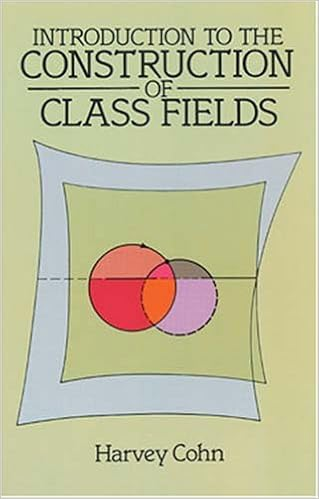### Book 3: Introduction to the Construction of Class Fields

Author: Harvey Cohn Category: Number Theory, Mathematics

Professor Cohn adopts a hypothetical Pythagorean issue to stimulate the development of a constructional introduction to classical field theory and modular function theory in this graduate-level textbook. With the introduction of accessible, affordable computer technology, the demand for constructional methods has rapidly surged. The introductory chapters give the inspiration and underlying knowledge of Riemann surface theory and elementary algebraic number theory that is required. Class Field theory is the next area in which the concepts and findings are deployed and expanded. Although the author stresses, for instance, the relevance of the subject to other areas of mathematics, the later chapters present more precise conclusions supported by in-depth arguments.

Buy this book at Amazon Book Store.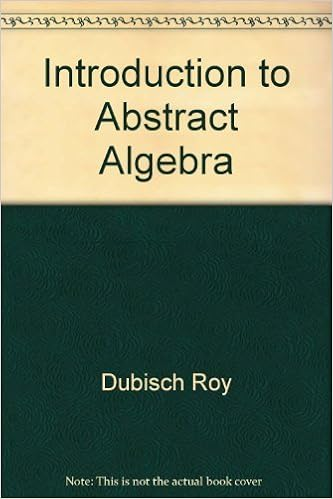### Book 4: Introduction to Abstract Algebra

Author: Roy Dubisch (Other Contributor) Category: Abstract Algebra

"Introduction to Abstract Algebra" does what it sets out to do. It explains in meticulous detail with a wealth of explanation examples the language and foundations of Abstract Algebra.

It commences with the foundations and nomenclature of Set Theory. Natural numbers are defined by the Peano postulates, from which logical development defines signed integers, equivalence classes, integral domains, rationals, groups, fields. Real numbers are defined by Cauchy sequences, as equivalence classes taken to the modulus of the set of null sequences.

The book goes on to explain rings, ideas, homomorphism, polynomials, and Euclid’s Algorithm is reached quite near the end.

Buy this book at Amazon Book Store.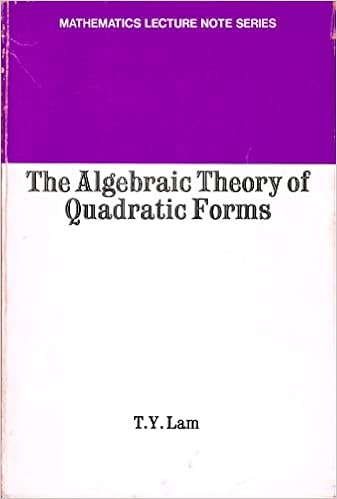### Book 5: Algebraic Theory of Quadratic Forms

Author: T.Y. Lam Category: Algebra, Mathematics

The book was developed by the author from two topics courses, offered to graduate students in mathematics. The only prerequisite is general knowledge of Algebra. Readers will find a full account of quadratic form theory over arbitrary fields. After covering Witt’s theory in one of the initial chapters, the author introduces the techniques of quaternion Algebras and of Clifford Algebras. Detailed theories of central simple graded Algebras, the Brauer-Wall group, and Clifford modules are made available in book form for the first time.

Other chapters include systematic treatments of local fields, global fields leading up to a concise discussion of Pfister forms, and the quadratic invariants of fields.

The text contains complete proofs as well as 150 problems. Solving these problems provides the reader with opportunities to test understanding of the text and to develop computational and deductive skills.

Buy this book at Amazon Book Store.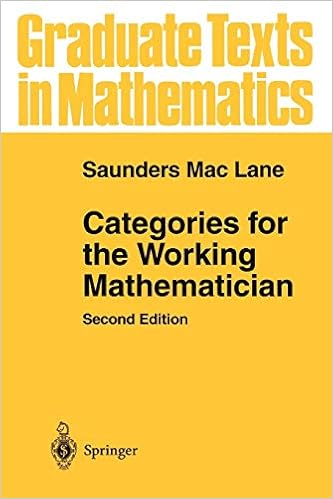### Book 6: Categories for the Working Mathematician (Graduate Texts in Mathematics, 5) 2nd ed. 1978. Softcover reprint of the original 2nd ed. 1978 Edition

Author: Saunders Mac Lane Category: Algebraic Geometry, Abstract Algebra, Geometry

The book underneath evaluation serves as an introduction to the theory of categories and, as the title implies, is written with the (no-nonsense) working mathematician in mind. As a result, it presents the principles and concepts of Category Theory in a broad context of common instances. This book introduces the fundamental notions of category, functor, natural transformation, and duality. The discussion then shifts to adjoint functors, which offer a description of universal constructions, an analysis of the representations of functors by sets of morphisms, and a method for manipulating direct and inverse limits.The remaining chapters, which include several applications of the fundamental existence theorem for adjoint functors, provide in-depth illustrations of these category ideas. According to Beck's theorem, the categories of algebraic systems build from particular adjoint-like facts. The book then moves on to the creation and use of Kan extensions after considering several applications. This second edition also features several revisions and additional chapters on subjects of current interest, such as braided and symmetric monoidal categories, along with the associated coherence theorems, as well as 2-categories and lately popular higher-dimensional categories.

The book continues to be a reliable resource for information on the theoretical underpinnings and a simple initial introduction to categories. It also contains many thought-provoking conversations and tasks.

Buy this book at Amazon Book Store.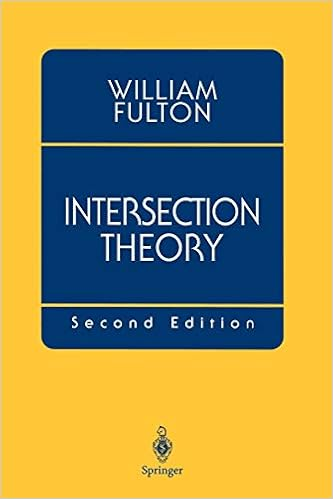### Book 7: Intersection Theory, 2nd Edition

Author: William Fulton Category: Algebraic Geometry, Number Theory, Geometry

Every algebraic geometer needs to read this book, which is a driving force in the field. It contains an excellent layout and practical information. It is a useful textbook, a valuable reference work, and a terrific place to start for future geometric study. The hardback version won the coveted Steele Prize for the largest exposition in 1996.

Intersection theory has played a significant role in mathematics for more than 200 years. It was applied to solve polynomial equations from the beginning of algebraic geometry to the successes of algebraic geometry. This book develops The theory's underlying principles, which also lists several traditional and contemporary applications.

Buy this book at Amazon Book Store.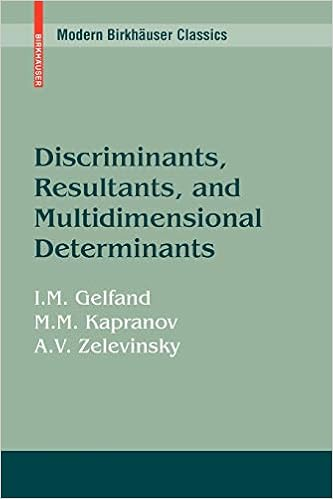### Book 8: Discriminants, Resultants, and Multidimensional Determinants (Modern Birkhäuser Classics) 1st Edition

Author: Israel M. Gelfand, Mikhail Kapranov, Andrei Zelevinsky Category: Algebraic Geometry, Abstract Algebra, Linear Algebra

In this work, The classic theory of resultants and discriminants is resurrected and massively enlarged. The majority of the significant recent findings from the book have already been published in more than a handful of the authors' collaborative papers. The book provides numerous examples that illustrate both the old and new outcomes of the theory, which complements these original papers perfectly. Over the past fifteen years, the book has evolved into a crucial timeless classic. Due to its innovative and motivating effects, it has sparked a straightforward avalanche of related research initiatives. This unique citation book continues to set the pace in numerous fields of current mathematics, from algebraic geometry and the general theory of hypergeometric functions to their most recent applications in mathematical physics, as evidenced by the slowly but surely growing number of quotations that appear to be flowing at all times. If you want to dive deep into modern algebra or look for a challenging problem to concentrate on that will advance society as a whole, then this is the best book for you.

Buy this book at Amazon Book Store.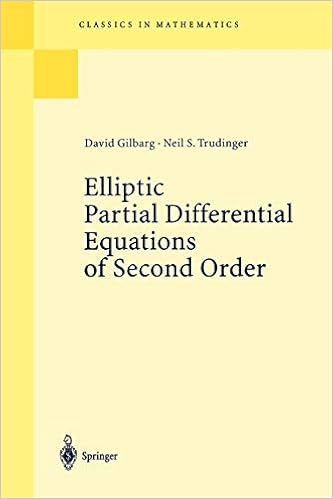### Book 9: Elliptic Partial Differential Equations of Second Order Reprint of the 2nd ed. Berlin Heidelberg New York 1983. Corr. 3rd printing 1998 Edition

Author: David Gilbarg, Neil S. Trudinger Category: Differential Equations, Pure Mathematics, Mathematics

This publication, which has withstood the test of time successfully, stands as a bibliographical monument to the theory of both theoretical and applied PDEs. It still plays a substantial and pivotal role in the discipline's ongoing research. Its purpose is to offer "the systematic development of the general theory of second-order quasilinear elliptic equations and the necessary linear theory." There are two parts to the book. The "linear theory" is covered in chapters 2 through 8, while the theory of quasilinear partial differential equations is covered in chapters 9 through 15. An Introduction (Chapter 1) that explains the key concepts and uses them as a roadmap throughout the book follows the 14 subsequent chapters. This paperback text on linear and quasilinear partial differential equations is in high demand among advanced students and professionals. You will find lots to consider and work on in the authors' book. You can use it as a textbook or reference, and there is even an epilogue that outlines the most recent findings. It's a great pleasure to read the book because the authors have brilliantly excelled in achieving their goals.

Buy this book at Amazon Book Store.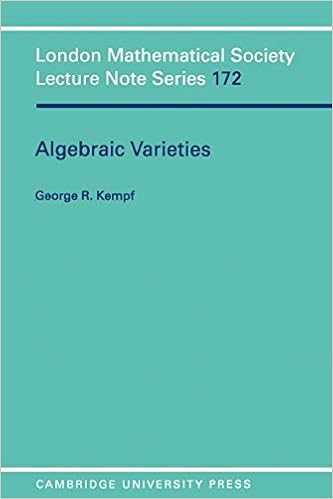### Book 10: Algebraic Varieties (London Mathematical Society Lecture Note Series, Series Number 172) 1st Edition

Author: G. Kempf Category: Analytic Geometry, Topology, Geometry

Professor Kempf has engagingly conveyed the subject in addition to employing geometric terminology. He introduces algebraic variety theory in this work by using the sheaf theory as a foundation. He offers a concise and comprehensive explanation of the subject by adopting this perspective, which will be easy to understand for all individuals who are not conversant with the algebraic varieties. Here, he offers a concise overview of cohomology theory on algebraic varieties, with applications to curves and surfaces via the Riemann-Roch theorem. Before demonstrating the connection with Cech theory, he could also compute using derived functor cohomology, which he does in this instance. He develops his unique proof of the Riemann-Roch theorem as a result of his investigation into how Galois might have arrived at the conclusions regarding abelian integrals that he describes in his renowned letter from a memoir that was reportedly destroyed. He has such a thorough understanding of his subject that he presents even the most complex ideas straightforwardly. Therefore, this book should be taken into account by graduate students who are interested in algebraic geometry.

Buy this book at Amazon Book Store.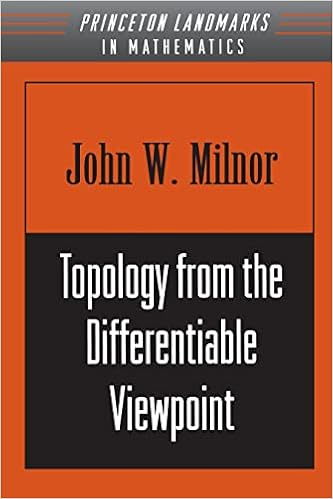### Book 11: Topology from the Differentiable Viewpoint Rev ed. Edition

Author: John Willard Milnor Category: Algebraic Geometry, Topology, Geometry

John Milnor, a renowned mathematician, introduces one of the most crucial topics in modern mathematics in this attractive work that is both simple and concise. He starts with fundamental ideas like diffeomorphisms and smooth manifolds, then moves on to tangent spaces, directed manifolds, and vector fields.The author also talks about Pontryagin construction, along with other significant concepts like homotopy and a map's index number. He gives examples of the proofs of Sard and Hopf's theorem. This book's primary objective is to expose readers to mathematics as a field of study, even though it covers some excellent themes. In broad strokes, it illustrates how a mathematical theory is developed—in this instance, Differential Topology—and, subsequently, what mathematicians do and take into account.Nowadays, many people only view mathematics as a helpful instrument, similar to a spreadsheet or a toaster oven. We disregard its capacity to broaden our minds and frame the unfathomable. This book serves as a reminder and demonstrates why mathematics is the reigning scientific field.Therefore,it should be picked up by anyone who wants to understand more about mathematics as a discipline rather than merely learn basic math.

Buy this book at Amazon Book Store.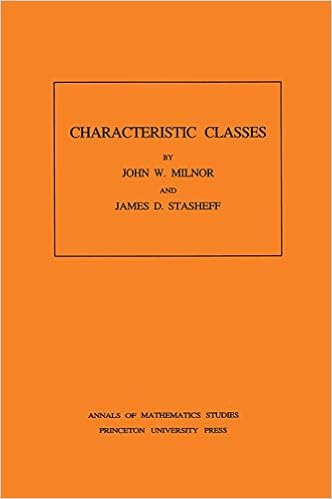### Book 12: Characteristic Classes. (AM-76) First Edition

Author: John Milnor, James D. Stasheff, Category: Differential Geometry, Topology, Algebraic Geometry

The concept of characteristic classes unites several disciplines, including fiber bundle theory, cohomology, differential topology, and differential and algebraic geometry. In light of this, it serves as a fundamental and crucial tool in the differentiable manifolds. The authors of this volume offer a complete introduction to characteristic classes with in-depth analyses of the Stiefel-Whitney, Chern, Pontrjagin, and Euler classes. Three appendices cover the principles of cohomology theory, the differential forms method of characterizing classes and a description of Bernoulli numbers. For publication, John Milnor's lecture notes underwent a thorough revision and editing process after being initially presented at Princeton University in 1957 and receiving extensive topological graduate student review since then.

Buy this book at Amazon Book Store.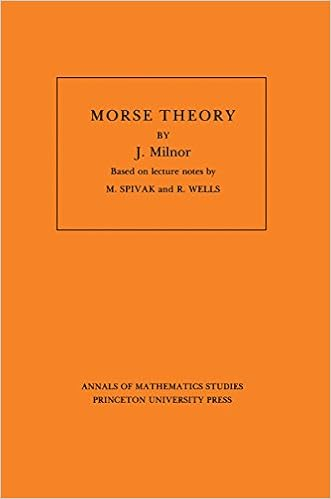### Book 13: Morse Theory (Annals of Mathematic Studies AM-51)

Author: John Milnor Category: Differential Geometry, Topology, Geometry

The presentation of Morse's idea by John Milnor has been the prominent book on the subject for more than 40 years. It is one of the most often cited books in mathematics. Mathematician Marston Morse created the Morse theory in the 1920s. The endeavor to understand an object's large-scale structure while having little or no information is a classical application of the Morse theory. Mechanical engineering, dynamic systems, and mathematical physics all deal with issues of this type. In a well-known publication, theoretical physicist Edward Witten built a connection between Morse theory and quantum field theory, which has garnered much attention for Morse theory over the past two decades. For his contributions to differential topology, Milnor received the Fields Medal. It was considered the mathematical equivalent of the Nobel Prize in 1962. He gained the National Medal of Science (1967) and the Steele Prize twice (1982 and 2004) by the American Mathematical Society in honor of his explanations of mathematical ideas in several scientific areas.

Buy this book at Amazon Book Store.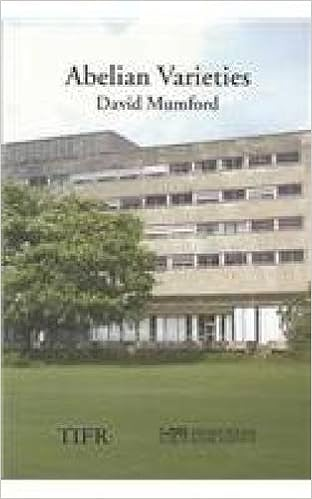### Book 14: Abelian Varieties (Tata Institute of Fundamental Research)

Author: David Mumford, C. P. Ramanujam, Yuri Manin Category: Algebra & Trigonometry, Algebra

It is a reproduction of the renowned 1970 book by David Mumford from the second, revised edition (1974). It provides a methodical overview of the key findings regarding abelian varieties. It explains analytical methods that can be used on the complex number ground field and offers scheme-theoretic methods for dealing with inseparable isogenies when the ground field displays a positive property. Moreover, this book contains independent evidence of the dual abelian variety's existence. It also explains how an abelian variety's ring of endomorphisms is structured. These appendices relate to the Mordell-Weil theorem and Tate's theorem on endomorphisms of abelian varieties over finite fields (by Yuri Manin). It is the only book that covers the topic thoroughly and sharply illustrates both The classical theory and The modern theory. For the foreseeable future, it will continue to be a classic that readers frequently revisit.

Buy this book at Amazon Book Store.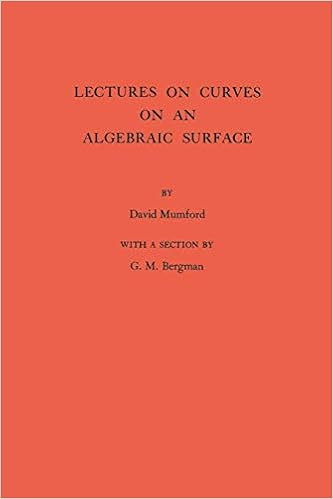### Book 15: Lectures on Curves on an Algebraic Surface. (AM-59), Volume 59 (Annals of Mathematics Studies) Kindle Edition

Author: David Mumford Category: Algebraic Geometry, Geometry

Using a non-singular projective algebraic curve constructed over an algebraically closed field of any characteristic, these lectures by Professor Mumford, delivered in 1963 and 1964 at Harvard, concentrate on the study of the features of families of algebraic curves. Grothendieck's strategies and methods—which have significantly altered the nature of algebraic geometry in recent years—are employed from the very beginning. As a result, the classical content is displayed from a fresh perspective.

Buy this book at Amazon Book Store.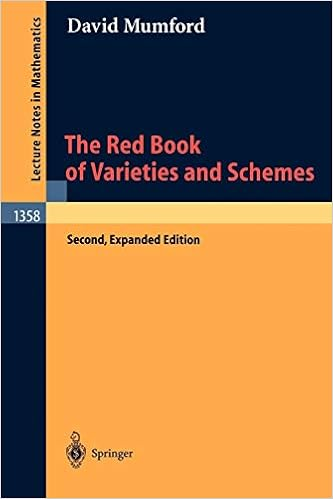### Book 16: The Red Book of Varieties and Schemes: Includes the Michigan Lectures (1974) on Curves and their Jacobians (Lecture Notes in Mathematics, 1358) 2nd exp. ed. 1999 Edition

Author: David Mumford (Author), E. Arbarello (Contributor) Category: Algebraic Geometry, Geometry, General

This second edition of a well-known and classic introduction to algebraic geometry aims to show how precisely the intuition of algebraic geometry is articulated in the fundamentally geometrical language of schemes. It combines the methods of commutative algebra with the geometric flavor of these fundamental algebraic geometry objects and provides a clear, understandable exposition of these concepts. It is designed for graduates and mathematicians in other professions who want to quickly learn about algebraic geometry. One of the most fascinating areas of algebraic geometry is covered in an appendix to this updated edition that provides an overview of curve theory, their moduli spaces, and their Jacobians.

This book is a must-read for anyone with an interest in algebraic geometry and a desire to learn about varieties, schemes, and their main problems.

Buy this book at Amazon Book Store.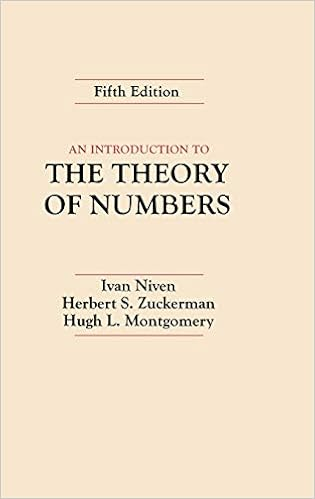### Book 17: An Introduction to the Theory of Numbers 5th Edition

Author: Ivan Niven, Herbert S. Zuckerman, Hugh L. Montgomery Category: Number Theory, Mathematics

One of the classic texts on number theory, by internationally renowned mathematicians, is now available in its fifth edition. For increased flexibility, chapters are essentially self-contained units. Some of the new additions include extended discussion of the binomial theorem, numerical calculating techniques, and a section on public key cryptography. It covers a remarkable set of issues.

Buy this book at Amazon Book Store.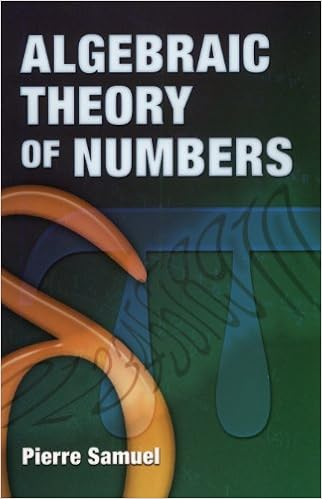### Book 18: Algebraic Theory of Numbers: Translated from the French by Allan J. Silberger (Dover Books on Mathematics) Kindle Edition

Author: Pierre Samuel Category: Number Theory, Pure Algebra

Students are introduced to new algebraic ideas as well as related ideas in algebraic number theory, such as groups, rings, fields, ideals, quotient rings, and quotient fields. This text covers the fundamentals, including the unit theorem, the finiteness of the class number, and Hilbert ramification theory. It also discusses divisibility theory in principal ideal domains.

Buy this book at Amazon Book Store.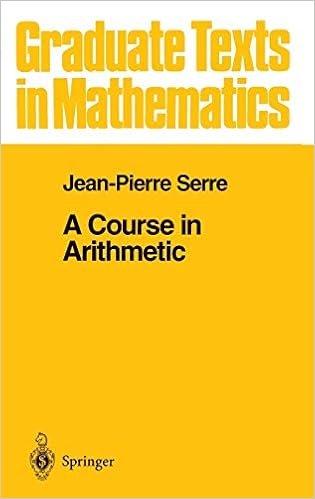### Book 19: A Course in Arithmetic (Graduate Texts in Mathematics, Vol. 7) (Graduate Texts in Mathematics, 7) 1st Corrected ed. 1973. Corr. 3rd printing 1996 Edition

Author: J-P. Serre Category: Number Theory, Mathematics, Schools & Teaching

The first one is entirely algebraic. Its goal is to categorize quadratic forms across the field of rational numbers (Hasse-Minkowski theorem). Chapter IV contains the accomplishment. Preliminaries like the quadratic reciprocity rule, p-adic fields, and Hilbert symbols are covered in the first three chapters.

The findings from Chapter IV are used for integral quadratic forms with discriminant ± I in Chapter V. Modular functions, differential topology, and finite groups are only a few examples of different questions that use these forms. Chapters VI and VII in the second portion employ "analytic" techniques (holomorphic functions). The Dirichlet "theorem on arithmetic progressions," which is substantially used in the first section, is proved in Chapter VI (Chapter Ill, no. 2.2). Modular forms, and specifically theta functions, are the subject of Chapter VII. Recurring here are a few of the quadratic forms from Chapter V. Thus, you should already have a thorough understanding of algebra and classical number theory before starting this book because it will broaden your perspectives.

Buy this book at Amazon Book Store.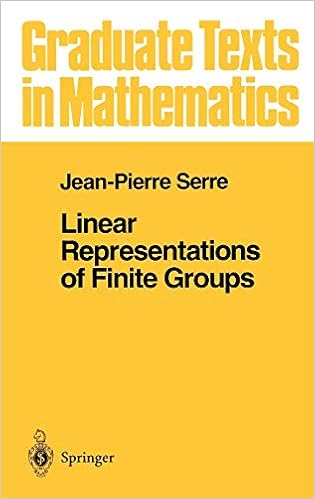### Book 20: Linear Representations of Finite Groups (Graduate Texts in Mathematics, 42) 1st ed. 1977. Corr. 5th printing 1996 Edition

Author: Jean-Pierre Serre, Leonhard L. Scott Category: Finite Mathematics, Group Theory, Abstract Algebra

There are three sections to this book, each with a somewhat different level and goal. The first section was initially created for quantum chemists. It explains how characters and linear representations correspond thanks to Frobenius. The second section is a lecture delivered in 1966 to Ecole Normale second-year students. In this way, it concludes the first section. An introduction to Brauer's theory is also provided in the third section.Serre's work acts as a superb introduction to representations for various audiences. The explanations in the exercises are as clear-cut and elegant as they always are with Serre, and they provide a plethora of essential information that would be hard to find otherwise. It is strongly recommended for both professionals and non-professionals.

Buy this book at Amazon Book Store.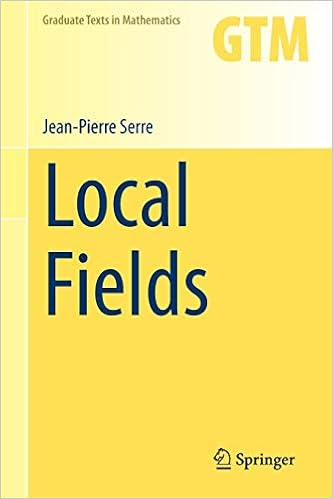### Book 21: Local Fields (Graduate Texts in Mathematics) Softcover reprint of the original 1st ed. 1979 Edition

Author: Jean-Pierre Serre, Marvin J. Greenberg (Translator) Category: Algebra & Trigonometry, Algebra

The purpose of this dissertation is to introduce local class field theory from a cohomological perspective, using the methodology developed by Hochschild and improved by Artin-Tate. This theory focuses primarily on abelian extensions of local (i.e., complete for a discrete valuation) fields with finite residue fields. One component of "localization" is the completion of an algebraic number field, for instance, to obtain these fields. "Parts" are created from the chapters. There are three introductory sections: the general theory of local fields is covered in the first two, and group cosmology is covered in the third. The fourth section covers the entire local class field theory. An explanation of what each of these four parts will contain is provided below: The first section provides fundamental concepts and findings on discrete valuation rings, Dedekind domains (which represent their "globalization"), and the completion process. For this section, it is necessary to have a basic understanding of algebra and topology, which can be obtained, for example, in Bourbaki. The topic of the second section is ramification phenomena (different, discriminant, ramification groups, and Artin representation). No assumptions are made regarding the residue fields in this section, just like in the first section. The "norm" map is investigated in this context, and the findings are presented in terms of "additive polynomials" and "multiplicative polynomials" rather than algebraic geometry because doing so would have taken one too far off course.

Buy this book at Amazon Book Store.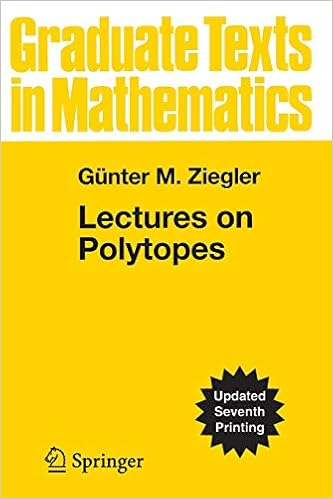### Book 22: Lectures on Polytopes (Graduate Texts in Mathematics, 152)

Author: Günter M. Ziegler Category: Algebraic Geometry, Geometry, Education

These lectures, which are based on a graduate course at the Technische Universität Berlin, contain a plethora of information on the contemporary theory of convex polytopes. For the majority of the theorems, the straightforward exposition includes countless instances and extensive evidence. It quickly transfers the reader from the fundamentals to the subjects of the current study with only linear algebra as a prerequisite. Basic information about polytopes is introduced in the lectures, with an emphasis on the techniques that produce the findings. Discussions of significant examples and beautiful constructions are also covered, and with the enthusiasm of recent research in the field.Both scholars and students will find them stimulating and fun to read.

Buy this book at Amazon Book Store.